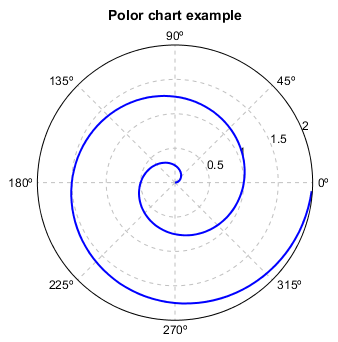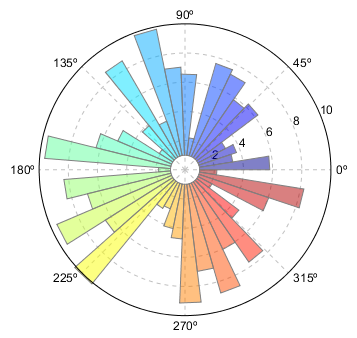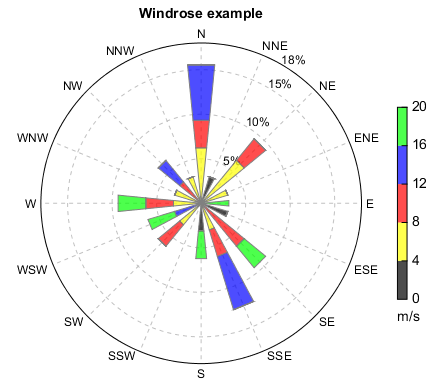# MeteoInfo 1.4.2 was released (2017-3-27)¶

Polar axes was supported to plot polar chart and windrose chart.

Plot a line in a polar axes

r = arange(0, 2, 0.01)
theta = 2 * pi * r

ax = axes(position=[0.1,0.1,0.8,0.8], polar=True)
plot(theta, r, color='b', linewidth=2)
title('Polor chart example')
antialias(True)Plot bars in a polar axes

# Compute pie slices
N = 40
theta = linspace(0.0, 2 * pi, N, endpoint=False)
radii = 10 * random.rand(N)
cols = makecolors(N, alpha=0.5)

axes(polar=True)
bars = bar(theta, radii, theta-theta, bottom=1, color=cols, edgecolor='gray')
antialias(True)Plot windrose chart

fn = r'D:\Temp\ascii\windrose.txt'
ncol = numasciicol(fn)
nrow = numasciirow(fn)